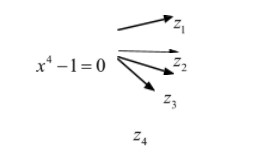QuestionAnswers

# If the fourth roots of unity are ${z_1},{z_2},{z_3},{z_4}$ then ${z_1}^2 + {z_2}^2 + {z_3}^2 + {z_4}^2$ is equal toA.$1$B.$0$C.$i$D.None of these

Hint :- Make use of the concept of fourth roots of unity and solve this
Fourth roots of Unity
Properties of Four Fourth Roots of Unity
a. Sum of all the four fourth roots of unity is zero.
b. The real fourth roots of unity are additive
Inverse of each other.
c. Both the complex / imaginary Fourth roots of
unity are conjugate for each other
d. Product of all the Fourth roots of unity is –

Complete step by step by solution
Let $x$ be the four fourth roots of $1$, if then we can write
$x = 4\sqrt 1$
We should write it
$x = {(1)^{\dfrac{1}{4}}}$
$\Rightarrow {x^4} = 1$
$\Rightarrow {x^4} - {1^4} = 0$
$\Rightarrow {({x^2})^2} - {({1^2})^2} = 0$
$[{a^2} - {b^2} = (a + b)(a - b)]$
Therefore,
$\Rightarrow ({x^2} - 1)({x^2} + 1) = 0$
Either,
$({x^2} - 1) = 0 or ({x^2} + 1) = 0$
${x^2} = 1 or {x^2} = - 1$
$x = \pm \sqrt 1 or x = \pm \sqrt { - 1}$
$x = \pm 1 or x = \pm i$
Now, the Four fourth roots are unity is $[1, - 1,i, - i]$
Step by step
(Image)
${z_1},{z_2},{z_3},{z_4}$ are roots of
${x^4} - 1 = 0$
$\therefore {z_1} + {z_2} + {z_3} + {z_4} = 0$
${z_1}{z_2} + {z_2}{z_3} + {z_3}{z_4} + {z_4}{z_1} + {z_1}{z_3} + {z_2}{z_4} = 0$
$\therefore {({z_1} + {z_2} + {z_3} + {z_4})^2} = \sum\limits_{}^{} {{z_1}^2}$
$\sum\limits_{i = 1}^4 {} \sum\limits_{i = 1}^4 {} {z_1}{z_i}$
$0 = {\sum {{z_1}} ^2} = 0$
$\therefore {\sum {{z_1}} ^2} = 0$B=$0$
Note–Complex numbers are the numbers which are expressed in the form of $a + ib$, where $i$ is an imaginary number called iota and has the value of $\sqrt { - 1}$.